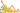# Loan Calculator

## Calculate Loan

Loan Amount

\$

Interest Rate

%

How many Years

years### Loan Calculator

Calculate Your Monthly Payment of Loan, How many Your Interest Paid on Loan, and the Total Loan Cost.
Loan calculators are tools that enable users to determine their monthly payment, total interest paid on the loan, and total loan cost. Just by adding loan amount, interest rate, and how many years.